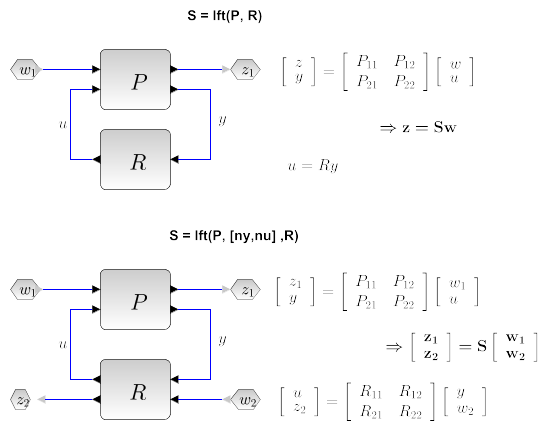Scilab Home page | Wiki | Bug tracker | Forge | Mailing list archives | ATOMS | File exchange
Please login or create an account
Change language to: Français - Português - 日本語 - Русский

Please note that the recommended version of Scilab is 6.1.0. This page might be outdated.
See the recommended documentation of this function

# lft

linear fractional transformation

### Syntax

```S=lft(P,R)
[S,s]=lft(P,p,R [,r])```

### Arguments

P

linear system (in state space or transfer function representation) or a simple gain, the ``augmented'' plant, implicitly partitioned into four blocks (two input ports and two output ports).

p

1x2 row vector, the dimensions of the `P_22` block (see below).

R

llinear system (in state space or transfer function representation) or a simple gain, implicitly partitioned into four blocks (two input ports and two output ports).

r

1x2 row vector, dimension of the `R_22` block . This argument should not be used. It is retained for compatibility with previous versions.

S

linear system, the linear fractional transform.

s

1x2 row vector, dimension of the `S_22` block (see below).

### Description

Linear fractional transform between two standard plants in state space form or in transfer form:Syntax `S=lft(P,R)`

Computes the linear fractional transform between the systems `P` and a controller `R`. The system `S` corresponds to the transferif `ny` and `nu` are respectively the number of inputs and outputs of `R`, one must have `size(P)>=[ny nu]`. The system returned is formally equivalent to

```i1 = 1:(\$-ny);j1=1:(\$-nu);
i2 = (\$-ny+1):\$;j1=(\$-nu+1):\$;
S = P(i1,j1) + P(i1,j2) * R * (eye() - P(i2,j2) * R) \P(i2,j1)```
Using `lft` instead of the code above avoids numerical problems and non minimal realization.

Syntax `[S,s]=lft(P,p,R)`

with `p= [ny,nu]` Forms the generalized (2 ports) lft of `P` and `R`.

`S` is the two-port interconnected plant, which correspond to the transfer:`s` is the dimension of the `22` block of `S`.

`P` and `R` can be PSSDs i.e. may admit a polynomial `D` matrix.

### Examples

```//simple feeback case

P=[1/%s, 1/(%s+1); 1/(%s+2),2/%s];
R= 1/(%s-1);
lft(P,R)

P(1,1)+P(1,2)*R*inv(1-P(2,2)*R)*P(2,1)   //Numerically dangerous!

lft(P,-1)

//Improper systems
W=[1,1;1,1/(%s^2+0.1*%s)];
K=tf2ss(1+1/%s+%s);////Improper (PID control)
lft(W,[1,1],K)```

### See also

• sensi — sensitivity functions
• augment — augmented plant
• feedback — feedback operation
• sysdiag — Create a block diagonal matrix from provided inputs or block diagonal system connection

### Comments

Add a comment:
Please login to comment this page.

 Report an issue << feedback Control Loop Disturbance Decoupling >>

 Scilab EnterprisesCopyright (c) 2011-2017 (Scilab Enterprises)Copyright (c) 1989-2012 (INRIA)Copyright (c) 1989-2007 (ENPC)with contributors Last updated:Tue Feb 14 15:02:44 CET 2017